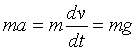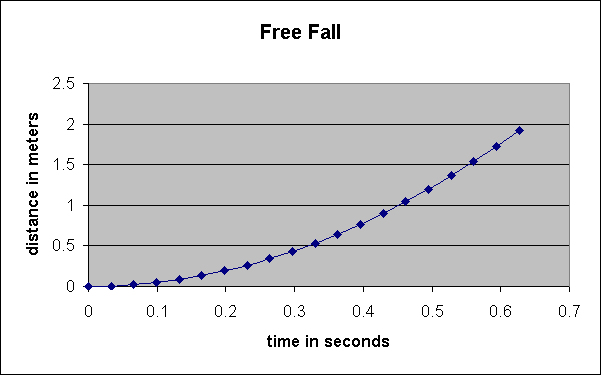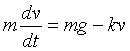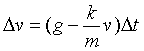HOME

Using Excel to Simulate Falling Motion

The plan here is to use Excel to plot velocity against time and distance against time for a falling ball, plotting a sequence of graphs starting with the simplest. (I'll write in bold things you should enter in the spreadsheet, although of course you don't need them to be in bold type in the spreadsheet.)

First, open up a spreadsheet and write Falling Motion under Gravity in cell A1.

In A3, write: We plot the position of a falling ball at time intervals delta_t.

(Maybe later we’ll figure out how to put D t, right now it’s too much trouble.)

In A5 write: delta_t =

Now click on B5, Insert/Name/Define. A box will come up, suggesting delta_t. Click OK.

If we’re trying to reproduce the motion observed on the video, the interval delta_t would be one-thirtieth of a second, so enter 0.0333 in B5.

We also will need the value of g, so write g = in A6, do the usual Insert/Name/Define, etc., let's take g = 10.

In B11 write "time", in C11 "velocity" and in D11 "distance". These cells are at the head of our table. (We’re leaving some space for later stuff.)

B12 will be the initial time, enter 0. B13 will be the time after the first time interval, so in B13 write " =B12 + delta_t". When you enter this, 0.0333 should appear in B13.

Now click on B13 so that it is surrounded by a square, with a small solid square covering the bottom right-hand corner of the frame around B13. Put the cursor on the small bottom right-hand corner square, the cursor will turn into a cross. Drag this down twenty rows. Excel will copy the formula down, and fill in the values in cells to B31, a list of times delta_t apart.

Zero Air Resistance

As a warm-up exercise, to get practice with using the spreadsheet, we consider the trivial case of zero air resistance. The equation of motion is than simplyor dv = gdt. The discretized version of this might be written delta_v = g.delta_t. This means that in a time interval delta_t, the velocity will increase by g times delta_t.

Taking the initial velocity to be zero, we enter 0 in C12, then in C13 put " =C12 + g*delta_t". Then, as with the times, we copy this formula down to C31, filling in the series of velocities corresponding to the times.

It has probably occurred to the reader at this point that we’re making heavy weather of a simple problem, we could have just put " =g*B12" in C12 and copied that formula down to give the same result. That’s quite true, but our more complicated approach generalizes easily to the case where there’s air resistance.

Now consider plotting distance fallen as a function of time. Put 0 in D12. We’ll measure distance downwards from the initial position. Now the distance fallen in an interval delta_t is equal to the average velocity during the time interval multiplied by delta_t. We take the average velocity to be half the sum of the initial and final velocities, which is exact for constant acceleration (but only an approximation otherwise).

So in D13 we enter: =D12 + 0.5*(C12 + C13)*delta_t, then we copy this down the column to D31.

Let us now plot a graph of distance fallen against time. Highlight cells B12 through B31, then, holding down Ctrl, highlight also cells D12 through D31. Now click the chart wizard (the little column graph on the toolbar), click XY Scatter, then pick the type that shows the points, but with a smooth curve going through them. Go to next, call the graph "Free Fall", say, put "time in seconds" on the x-axis, and "distance in meters" on the y-axis. Click Finish, and your chart should be on your sheet. You can move it or resize it if you wish. It should look like this:With the chart in place, you can now see how a ball falls on the moon by changing the value of g in B6 to 2. Of course, you can also change delta_t.

Air Resistance

Assuming first air resistance proportional to velocity, Newton’s Second Law is:where k is the strength of the air resistance. A discretized form of this equation would be:In contrast to the free fall case, this equation is not exact for finite D t. Obviously, the velocity change over a time interval will depend on how the velocity varies over that interval. If we choose time intervals sufficiently small that we can neglect the change in velocity in the interval compared with v, we can adapt the above formula by replacing v in it by the value of v at the beginning of the interval.

Before we go on, we have some new parameters to enter, m and k. We write m= in A7, k= in A8. Naming B7 and B8 m and k respectively, let’s put default values of m = 1 and k = 0.1.

Now, in C13 replace the formula =C12 + g*delta_t by =C12+(g – (k/m)*C12)*delta_t.

Copy this formula down to the end of the table.

At this point, it is also worth creating a graph of velocity over time. This graph makes it more evident that a terminal velocity is being approached. The two graphs, of position against time and velocity against time, can be arranged so that both are visible at the same time on the screen, along with the values of delta_t, g and k. It might be a good idea to turn off the autoscaling of the y-axis for the velocity, so right-click on the y-axis, click Format Axis, click Scale, and set Maximum = 10, disabling the autoscaling by clicking on the check box next to Maximum, so that the check disappears. This will make it more evident how the terminal velocity varies with air resistance.

Exercises

1. Take delta_t = 0.033, g = 10, m = 1, k = 0. The graphs should look very like those of the video experiment of dropping the ball. (Note that the curves won’t depend on mass as long as the air resistance coefficient k = 0). Now try k = 1, 2, 5 to see how terminal velocity is approached.

2. Taking k = 1, put delta_t = 0.5. This makes the approach to terminal velocity very clear. Now put k = 2. What does the curve look like? Then try k = 3, then k = 4. Do your curves correspond to physical reality? Obviously, the discretization more accurately reflects the true dynamics if delta_t is made smaller. Keeping k = 4, reduce delta_t to 0.25 then 0.1. What can you conclude?

3. Recall that for this case we know the exact answer for the velocity,We can plot this exact answer to find out how good our numerical approximation is. So in E11 write "exact", then in E12 enter the formula: =m*(g/k)*(1-EXP(-k*(B12/m))), and copy it down the column. Now, select B12 to C31, then hold Ctrl and select E12 through E31, then click Chartwizard. The exact answer and the numerically computed answer now appear on the same graph. By experimenting with delta_t and k, it becomes evident when the numerical analysis can be trusted. Keep this graph in place for the next exercise.

4. Now let’s see how things change when the drag force is proportional to the square of the velocity.

First, save your spreadsheet as FallingBall1, say, then click Save As and re-save it as FallingBall2.

Now, in FallingBall2, in A9, write b=, then insert the name b in B9, then insert a numerical value, begin with the same one as in B8. Now replace the formula for velocity in C13 by: =C12+(g-(b/m)*C12*C12)*delta_t, and copy it down the column.

Look at the graph you have comparing this velocity squared drag force with the exact proportional to velocity drag force. Of course, they have different terminal velocities. Adjust b until the terminal velocities are about the same. You may need to adjust delta_t a bit to be clear terminal velocity is reached, but be careful!

The two curves are noticeably different. Give a physical explanation of the differences you see.

5. Nonzero initial velocity. Go back to fallingBall1, the sheet with air resistance proportional to velocity. Let us consider the case of nonzero initial velocity, such as a ball thrown downwards (or an asteroid crashing down?). In A10 write v_init= , insert this name in B10, along with 0 as the first value, then in C12 write "=v_init". That’s all you have to do. Now experiment with different values of v_init and k. See what happens if v_init is greater than the terminal velocity, or if v_init is negative, and think through physical explanations of what’s going on in these situations.

6. Throwing the ball upwards with velocity squared drag force. In the case of drag force linear in velocity given above, we got a sensible physical picture even when the initial velocity was upwards instead of downwards. However, when the force is proportional to the square of the velocity, we have to look at the formulas more carefully if the ball is initially thrown upwards. Can you see why? The answer is that the drag force is always a drag, that is, it opposes motion.

It is interesting to try out FallingBall2 with negative v_init anyway and see what happens. As v_init approaches the negative of the terminal velocity, everything goes crazy! Can you see why that is by looking at the equation?

OK, so we have to fix the equation so that the drag force always opposes motion. For the proportional to velocity case, we wrote the drag as –kv, so the force acted upwards when the ball was moving downwards and the force acted downwards when the ball was moving upwards. However, when we write a drag force –bv2, this is negative no matter what the sign of v. In other words, the way we’ve set it up, this force always acts upwards. This is of course unphysical—it implies that if the ball is thrown up fast, it will accelerate upwards! So our equation doesn’t reflect reality. The solution is to use Excel’s Sign function—this function equals +1 if it has a positive argument, -1 if it has a negative argument. So, in C13 we write:

=C12+(g-(SIGN(C12))*(b/m)*C12*C12)*delta_t

This will now give sensible answers, provided delta_t is sufficiently small that v doesn’t vary too much in one step. Actually, we’ve been unnecessarily restrictive in taking such a small spreadsheet. We could select the last row of the spreadsheet, then drag the formulas down 200 rows, say, using the little square in the bottom right-hand corner. We then need to fix up the graphs, or just create new ones. Choose the option without markers, just the lines. It is now possible to take delta_t much smaller and get a more precise picture.